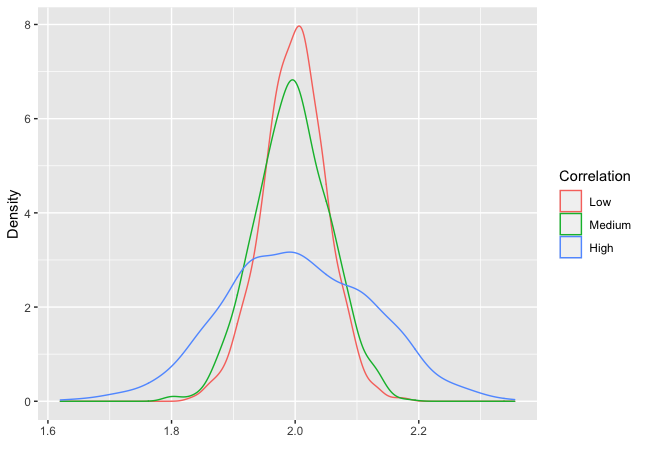# R code to illuatrate VIF in OLS

Remeber that the variance inflation factor (VIF) is given by

$\hat{var}(\beta_j)=\frac{s^2}{(n-1)\hat{var}(X_j)}\times\frac{1}{1-R^2_j}$

where $$R^2_j$$ is the $$R^2$$ from the regression of $$X_j$$ on the other covariates.

However, when first learning about VIF is can be hard to comprehend. To make it easier some illustrations are often nice.Below is some code to illustrate the variance inflation factor in R.

Tags:

Updated: